tsa

Time-synchronous signal average

Description

example

ta = tsa(x,fs,tp) returns a time-synchronous average of a signal x, sampled at a rate fs, on the pulse times specified in tp.

ta = tsa(x,t,tp) returns a time-synchronous average of x sampled at the time values stored in t.

example

ta = tsa(xt,tp) returns a time-synchronous average of a signal stored in the MATLAB® timetable xt.

example

ta = tsa(___,Name,Value) specifies additional options for any of the previous syntaxes using name-value pair arguments. For example, you can specify the number of tachometer pulses per shaft rotation or choose to average the signal in the time domain or the frequency domain.

[ta,t,p,rpm] = tsa(___) also returns t, a vector of sample times corresponding to ta; a vector p of phase values; and rpm, the constant rotational speed (in revolutions per minute) corresponding to ta.

tsa(___) with no output arguments plots the time-synchronous average signal and the time-domain signals corresponding to each signal segment in the current figure.

Examples

collapse all

Compute the time-synchronous average of a noisy sinusoid.

Generate a signal consisting of a sinusoid embedded in white Gaussian noise. The signal is sampled at 500 Hz for 20 seconds. Specify a sinusoid frequency of 10 Hz and a noise variance of 0.01. Plot one period of the signal.

fs = 500;
t = 0:1/fs:20-1/fs;

f0 = 10;
y = sin(2*pi*f0*t) + randn(size(t))/10;

plot(t,y)
xlim([0 1/f0])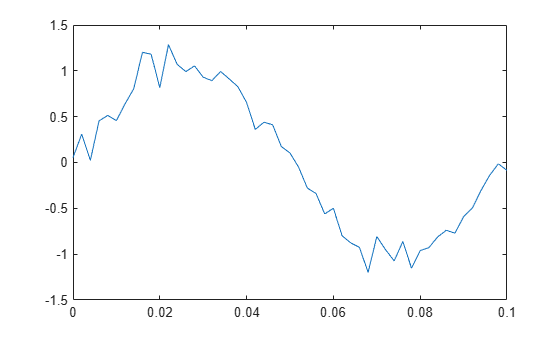Compute the time-synchronous average of the signal. For the synchronizing signal, use a set of pulses with the same period as the sinusoid. Use tsa without output arguments to display the result.

tPulse = 0:1/f0:max(t);

tsa(y,fs,tPulse)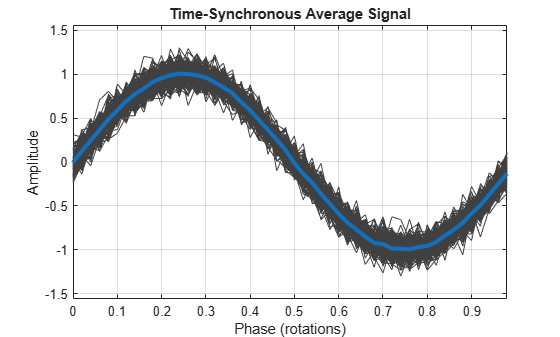Generate a signal that consists of an exponentially damped quadratic chirp. The signal is sampled at 1 kHz for 2 seconds. The chirp has an initial frequency of 2 Hz that increases to 28 Hz after the first second. The damping has a characteristic time of 1/2 second. Plot the signal.

fs = 1e3;
t = 0:1/fs:2;

plot(t,x)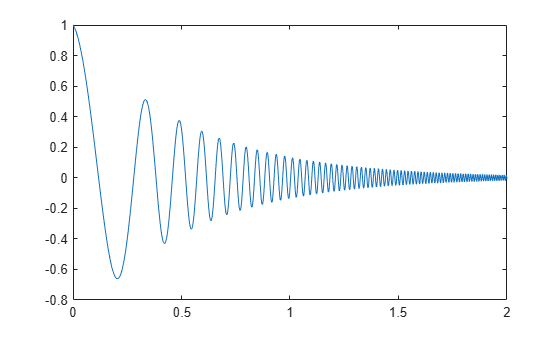Create a duration array using the time vector. Construct a timetable with the duration array and the signal. Determine the pulse times using the locations of the signal peaks. Display the time-synchronous average.

ts = seconds(t)';
tx = timetable(ts,x);

[~,lc] = findpeaks(x,t);
tsa(tx,lc)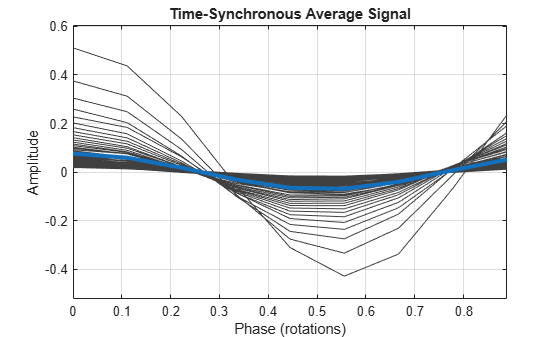Compute the time-synchronous average. View the types of the output arguments. The sample times are stored in a duration array.

[xta,xt,xp,xrpm] = tsa(tx,lc);
whos x*
Name         Size            Bytes  Class        Attributes

x         2001x1             16008  double
xp           9x1              1135  timetable
xrpm         1x1                 8  double
xt           9x1                74  duration
xta          9x1              1133  timetable

Convert the duration array to a datetime vector. Construct a timetable using the datetime vector and the signal. Compute the time-synchronous average, but now average over sets of 15 rotations.

View the types of the output arguments. The sample times are again stored in a duration array, even though the input timetable used a datetime vector.

dtb = datetime(datevec(ts));
dtt = timetable(dtb,x);

nr = 15;
tsa(dtt,lc,'NumRotations',nr)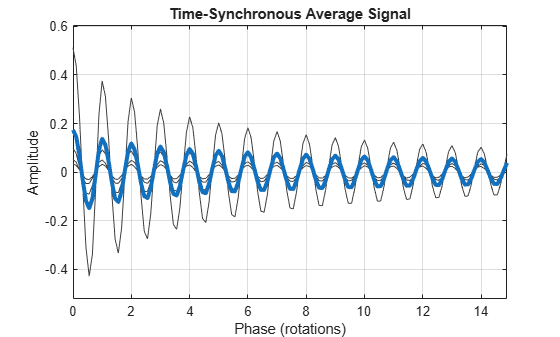[dta,dt,dp,drpm] = tsa(dtt,lc,'NumRotations',nr);
whos d*
Name         Size            Bytes  Class        Attributes

dp         135x1              3151  timetable
drpm         1x1                 8  double
dt         135x1              1082  duration
dta        135x1              3149  timetable
dtb       2001x1             32016  datetime
dtt       2001x1             49001  timetable

Compute the time-synchronous average of the position of a fan blade as it slows down after switchoff.

A desk fan spinning at 2400 rpm is turned off. Air resistance (with a negligible contribution from bearing friction) causes the fan rotor to stop in approximately 5 seconds. A high-speed camera measures the x-coordinate of one of the fan blades at a rate of 1 kHz.

fs = 1000;
t = 0:1/fs:5-1/fs;

rpm0 = 2400;

Idealize the fan blade as a point mass circling the rotor center at a radius of 10 cm. The blade experiences a drag force proportional to speed, resulting in the following expression for the phase angle:

$\varphi =2\pi {\mathit{f}}_{0}\mathit{T}\left(1-{\mathit{e}}^{-\mathit{t}/\mathit{T}}\right),$

where ${\mathit{f}}_{0}$ is the initial frequency and $\mathit{T}=0.75$ second is the decay time.

a = 0.1;
f0 = rpm0/60;
T = 0.75;

phi = 2*pi*f0*T*(1-exp(-t/T));

Compute and plot the x- and y-coordinates. Add white Gaussian noise.

x = a*cos(phi) + randn(size(phi))/200;
y = a*sin(phi) + randn(size(phi))/200;

plot(t,x,t,y)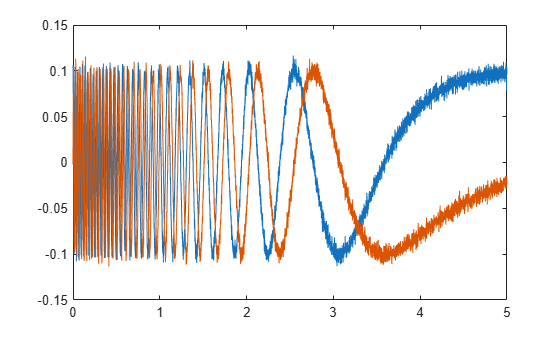Determine the synchronizing signal. Use the tachorpm function to find the pulse times. Limit the search to times before 2.5 seconds. Plot the rotational speed to see its exponential decay.

[rpm,~,tp] = tachorpm(x(t<2.5),fs);
tachorpm(x(t<2.5),fs)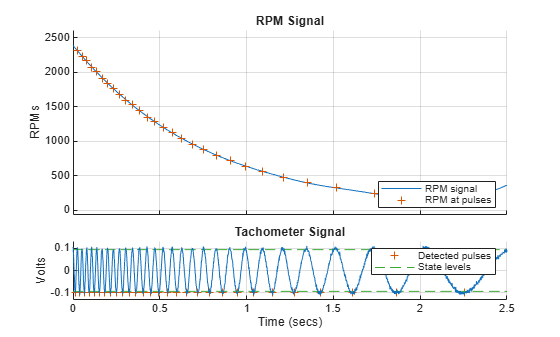Compute and plot the time-synchronous average signal, which corresponds to a period of a sinusoid. Perform the averaging in the frequency domain.

clf
tsa(x,fs,tp,'Method','fft')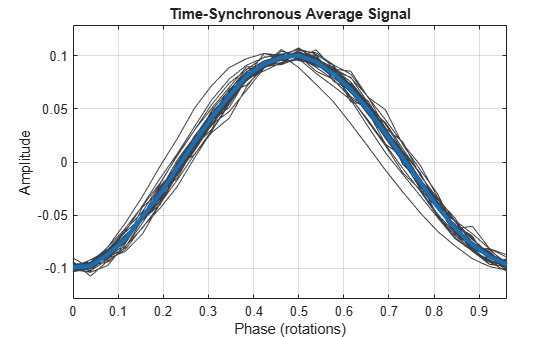Input Arguments

collapse all

Input signal, specified as a vector.

Example: cos(pi/4*(0:159))+randn(1,160) is a single-channel, row-vector signal.

Data Types: single | double

Sample rate, specified as a positive scalar.

Data Types: single | double

Pulse times, specified as a scalar or a vector.

• Scalar — a constant time interval over which rotations occur.

• Vector — nonnegative, strictly increasing instants that define constant rotational phase.

Use tachorpm to extract tachometer pulse times from a tachometer signal.

Data Types: single | double

Sample times, specified as a vector, a duration scalar, or a duration array.

• Scalar — the time interval between consecutive samples of x.

• Vector or duration array — the time instant corresponding to each element of x.

Data Types: single | double | duration

Input timetable. xt must contain increasing finite row times and only one variable consisting of a vector.

If a timetable has missing or duplicate time points, you can fix it using the tips in Clean Timetable with Missing, Duplicate, or Nonuniform Times.

Example: timetable(seconds(0:4)',randn(5,2)) specifies a two-channel, random variable sampled at 1 Hz for 4 seconds.

Data Types: single | double

Name-Value Arguments

Specify optional comma-separated pairs of Name,Value arguments. Name is the argument name and Value is the corresponding value. Name must appear inside quotes. You can specify several name and value pair arguments in any order as Name1,Value1,...,NameN,ValueN.

Example: 'Method','pchip','ResampleFactor',10 specifies that the signal is to be upsampled by a factor of 10 and averaged in the time domain using piecewise-cubic Hermite interpolation.

Interpolation scheme, specified as the comma-separated pair consisting of 'InterpMethod' and one of these values:

• 'linear' — Perform linear interpolation and average in the time domain.

• 'spline' — Perform cubic spline interpolation and average in the time domain.

• 'pchip' — Perform piecewise-cubic Hermite interpolation and average in the time domain.

• 'fft' — Perform frequency-domain averaging.

Number of shaft rotations in ta, specified as the comma-separated pair consisting of 'NumRotations' and a positive integer scalar.

Data Types: single | double

Number of time instants per shaft rotation, specified as the comma-separated pair consisting of 'PulsesPerRotation' and a positive scalar.

Data Types: single | double

Factor by which to increase the sample rate, specified as the comma-separated pair consisting of 'ResampleFactor' and a positive integer scalar.

Data Types: single | double

Output Arguments

collapse all

Time-synchronous signal average, returned as a vector or timetable. If the input to tsa is a timetable, then ta is also a timetable.

• If the input timetable stores the time values as a duration array, then the time values of ta are also a duration array.

• If the input timetable stores the time values as a datetime array, then the time values of ta are a duration array expressed in seconds.

Sample times, returned as a vector or duration array.

• If the input to tsa is a timetable that stores time values as a duration array, then t has the same format as the input time values.

• If the input to tsa is a timetable that stores time values as a datetime array, then t is a duration vector expressed in seconds.

• If the input to tsa is a numeric vector and the input sample times t are stored in a duration scalar or a duration array, then t is a duration array with the same units as the input t.

Phase values, returned as a vector or timetable expressed in revolutions.

If the input to tsa is a timetable, then p is also a timetable. p has the same values as the time values of ta.

Constant rotational speed, returned as a scalar expressed in revolutions per minute.

Algorithms

Given an input signal, a sample rate, and a set of tachometer pulses, tsa performs these steps:

1. Determines cycle start and end times based on the tachometer pulses and the value specified for 'PulsesPerRotation'.

2. Resamples the input signal based on the value specified for 'ResampleFactor'.

3. Averages the resampled signal based on the option specified for 'Method'.

• If 'Method' is set to 'fft', the function:

1. Breaks the signal into segments corresponding to the different cycles.

2. Computes the discrete Fourier transform of each segment.

3. Truncates the longer transforms so all transforms have the same length.

4. Averages the spectra.

5. Computes the inverse discrete Fourier transform of the average to convert it to the time domain.

• If 'Method' is set to one of the time-domain methods, the function:

1. Using the specified method, interpolates the signal onto grids of equally spaced samples corresponding to the different cycles.

2. Concatenates the resampled signal segments based on the value specified for 'NumRotations'.

3. Computes the average of all the segments.

 Bechhoefer, Eric, and Michael Kingsley. "A Review of Time-Synchronous Average Algorithms." Proceedings of the Annual Conference of the Prognostics and Health Management Society, San Diego, CA, September-October, 2009.